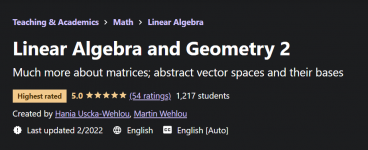#### Ruchika oberoi

Staff member### What you’ll learn​

• How to solve problems in linear algebra and geometry (illustrated with 153 solved problems) and why these methods work.
• Important concepts concerning vector spaces, such as basis, dimension, coordinates, and subspaces.
• Linear combinations, linear dependence and independence in various vector spaces, and how to interpret them geometrically in R2 and R3.
• How to recalculate coordinates from one basis to another, both with help of transition matrices and by solving systems of equations.
• Row space, columns space and nullspace for matrices, and about usage of these concepts for solving various types of problems.
• Linear transformations: different ways of looking at them (as matrix transformations, as transformations preserving linear combinations).
• How to compose linear transformations and how to compute their standard matrices in different bases; compute the kernel and the image for transformations.
• Understand the connection between matrices and linear transformations, and see various concepts in accordance with this connection.
• Work with various geometrical transformations in R2 and R3, be able to compute their matrices and explain how these transformations work.
• Understand the concept of isometry and be able to give some examples, and formulate their connection with orthogonal matrices.
• Transform any given basis for a subspace of Rn into an orthonormal basis of the same subspace with help of Gram-Schmidt Process.
• Compute eigenvalues, eigenvectors, and eigenspaces for a given matrix, and give geometrical interpretations of these concepts.
• Determine whether a given matrix is diagonalizable or not, and perform its diagonalization if it is.
• Understand the relationship between diagonalizability and dimensions of eigenspaces for a matrix.
• Use diagonalization for problem solving involving computing the powers of square matrices, and motivate why this method works.
• Be able to formulate and use The Invertible Matrix Theorem and recognise the situations which are suitable for the determinant test (and which are not).
• Use Wronskian to determine whether a set of smooth functions is linearly independent or not; be able to compute Vandermonde determinant.
• Work with various vector spaces, for example with Rn, the space of all n-by-m matrices, the space of polynomials, the space of smooth functions.

### Requirements​

• Linear Algebra and Geometry 1 (systems of equations, matrices and determinants, vectors and their products, analytic geometry of lines and planes)
• High-school and college mathematics (mainly arithmetic, some trigonometry, polynomials)
• Some basic calculus (used in some examples)
• Basic knowledge of complex numbers (used in an example)

### Who this course is for:​

• University and college engineering

Replies
0
Views
26
Replies
0
Views
31
Replies
0
Views
29
Replies
0
Views
27
Replies
0
Views
28